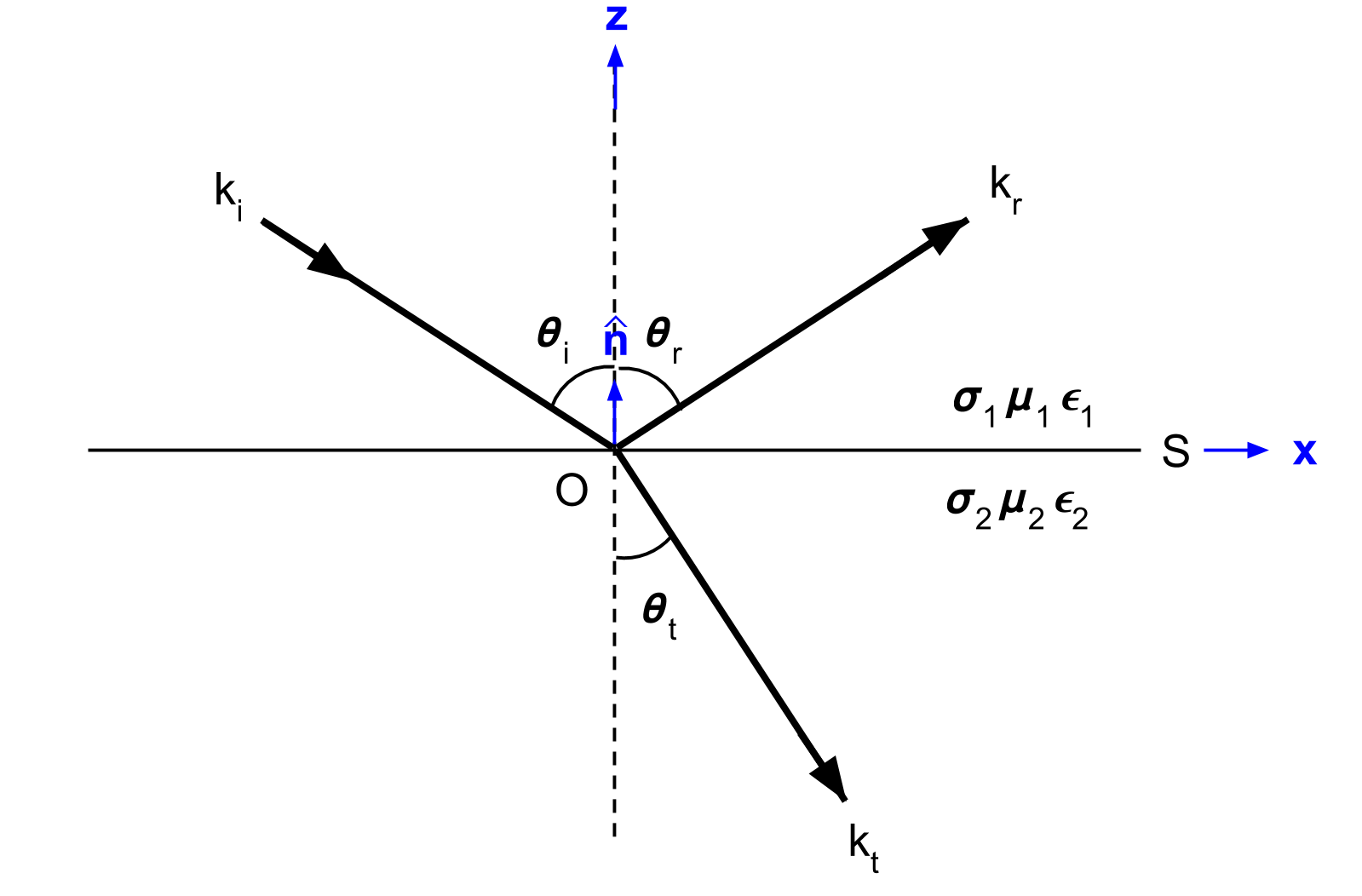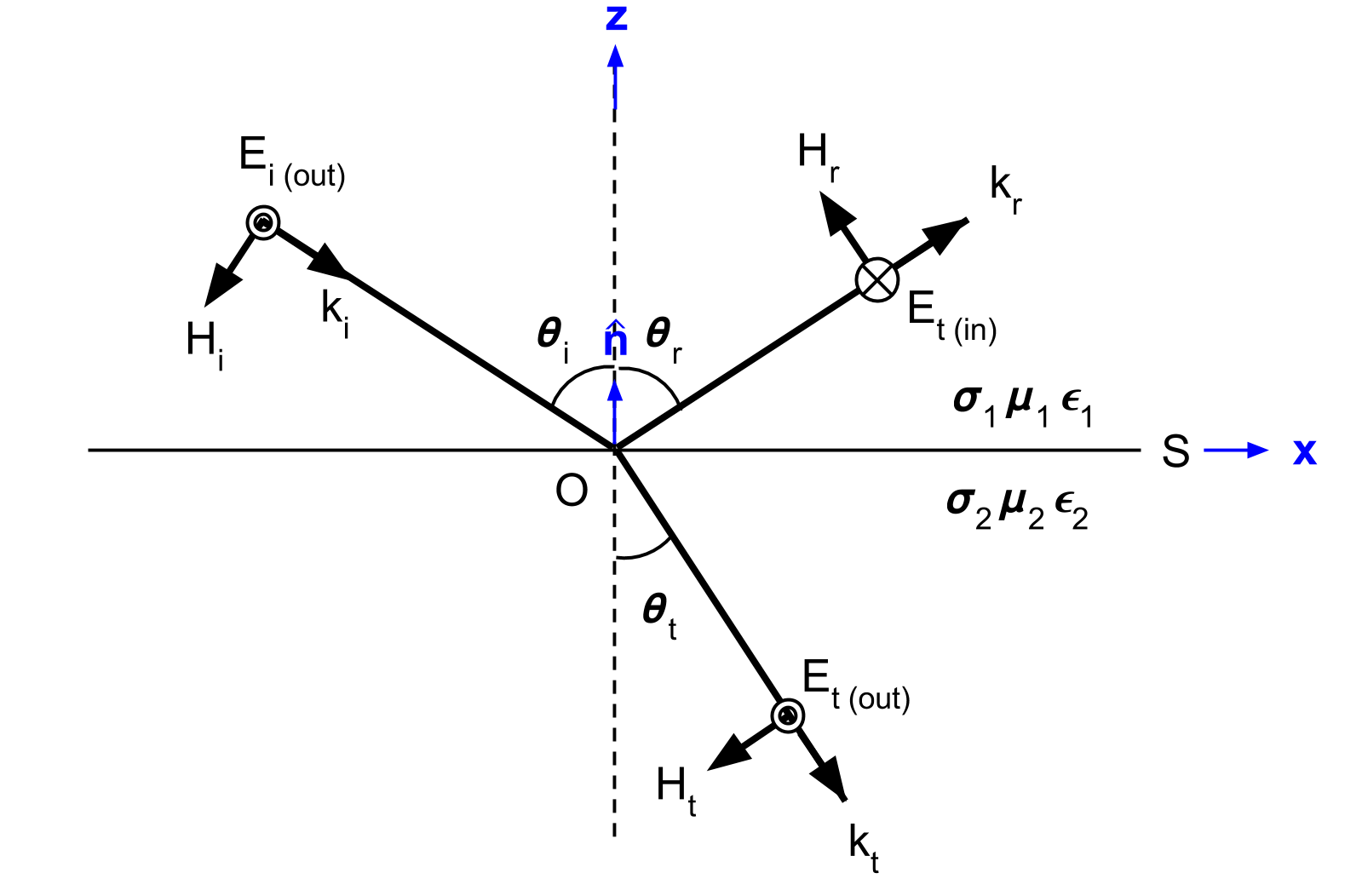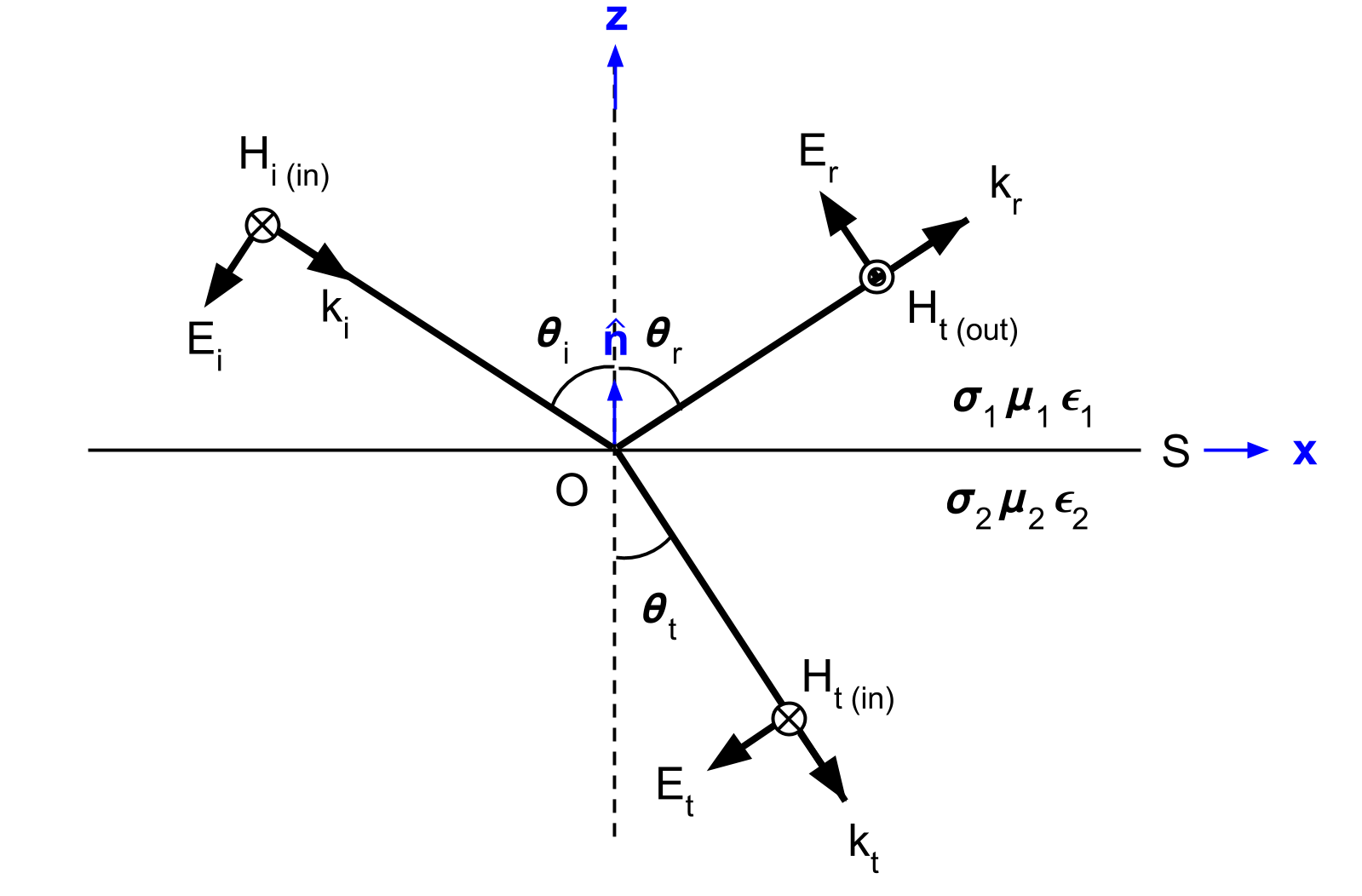In [ ]:
from ipywidgets import *
from geoscilabs.em.Reflection import PowerReflection


# Purpose¶

Electromagnetic waves are reflected and refracted at a plane interface separating media of different electrical properties. Essential items to understand here are:

• What are TE and TM modes for reflection, and refraction
• How reflection and refraction changes due to incidient angle and electrical parameters
• Total reflection
• Brewster angle

# Set up¶

A plane wave is reflected and refracted at a plane wave interface. Here $\mathbf{k}$ is wave propagation consant, which is a vector. We consider two different modes:

• Transverse electric (TE): No vertical electric field
• Transverse magnetic (TM): No vertical magnetic field## TE mode¶

Electric field ($\mathbf{E}$) is transverse to the plane of incidence (No vertical electric field).## TM mode¶

Magnetic field ($\mathbf{H}$) is transverse to the plane of incidence (No vertical electric field).# Reflection and Transmision app¶

## Parameters¶

• $\sigma_1$: Conductivity of the first layer (S/m)

• $\sigma_2$: Conductivity of the first layer (S/m)

• $f$ (Hz): Frequency (Hz)

• Type:

• Reflection: Transmission power as a function of incident angle
• Transmission: Transmission power as a function of incident angle
• Angle: relationship between $\theta_i$ and $\theta_t$
In [ ]:
Q = interact(PowerReflection
, sig1 = FloatText(value=0., description="$\sigma_1$")
, sig2 = FloatText(value=1e-2, description="$\sigma_2$")
, f = FloatText(value=6e5, description="$f$ (Hz)")
, Type = ToggleButtons(options=["Reflection", "Transmission", "Angle"])
)

In [ ]: